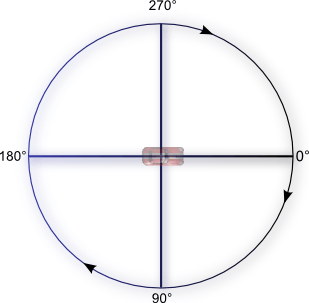# Common conversions

This category helps the user to do angle conversions and number to/from text conversions.

## Angle conversion

Angles can either be expressed in degrees or in radians. A circle describes an angle of 360 degrees or 2π radians. Similarly, a semicircle describes an angle of 180 degrees or π radians.

If you use trigonometric functions like the sine or the cosine, you will need to express the angles in radians. Use `ToRad` or `ToDeg` in numerical expressions to convert degrees to radians or radians to degrees.

Tip

Angles can be negative too. For example, -90 degrees is equal to 270 degrees.## Number to/from text conversions

For a string field, only a text can be filled in the field. Similarly, only a number can be filled in a text field.

To input a string in a numerical expression, you can convert it to number using the "Text > Number" expression. If the string input contains alphabetical characters or any other character than numeric characters, the expression will return "NaN", i.e. not a number.

To input a number in string expression, you can use the "Number > Text" expression. If the number used is greater than or equal to 1e+21, the expression returns its value in scientific notation.

## Reference

All actions, conditions and expressions are listed in conversions reference page.